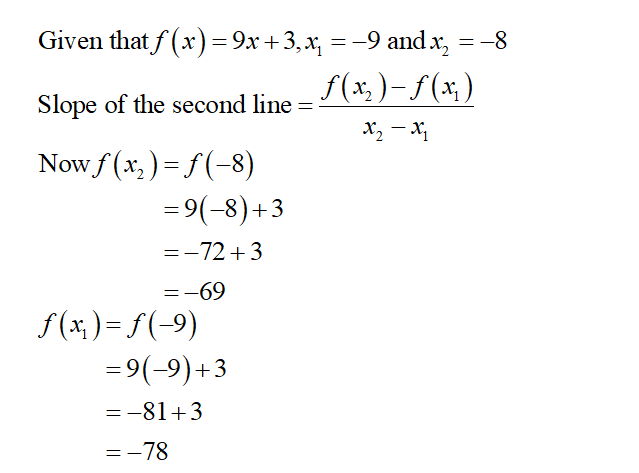# If f(x)=9x+3f(x)=9x+3, find the slope of the secant line between the values of x1=−9x1=-9 and x2=−8x2=-8.

Question
12 views

If f(x)=9x+3f(x)=9x+3, find the slope of the secant line between the values of x1=−9x1=-9 and x2=−8x2=-8.

check_circle

Step 1...

### Want to see the full answer?

See Solution

#### Want to see this answer and more?

Solutions are written by subject experts who are available 24/7. Questions are typically answered within 1 hour.*

See Solution
*Response times may vary by subject and question.
Tagged in

### Calculus